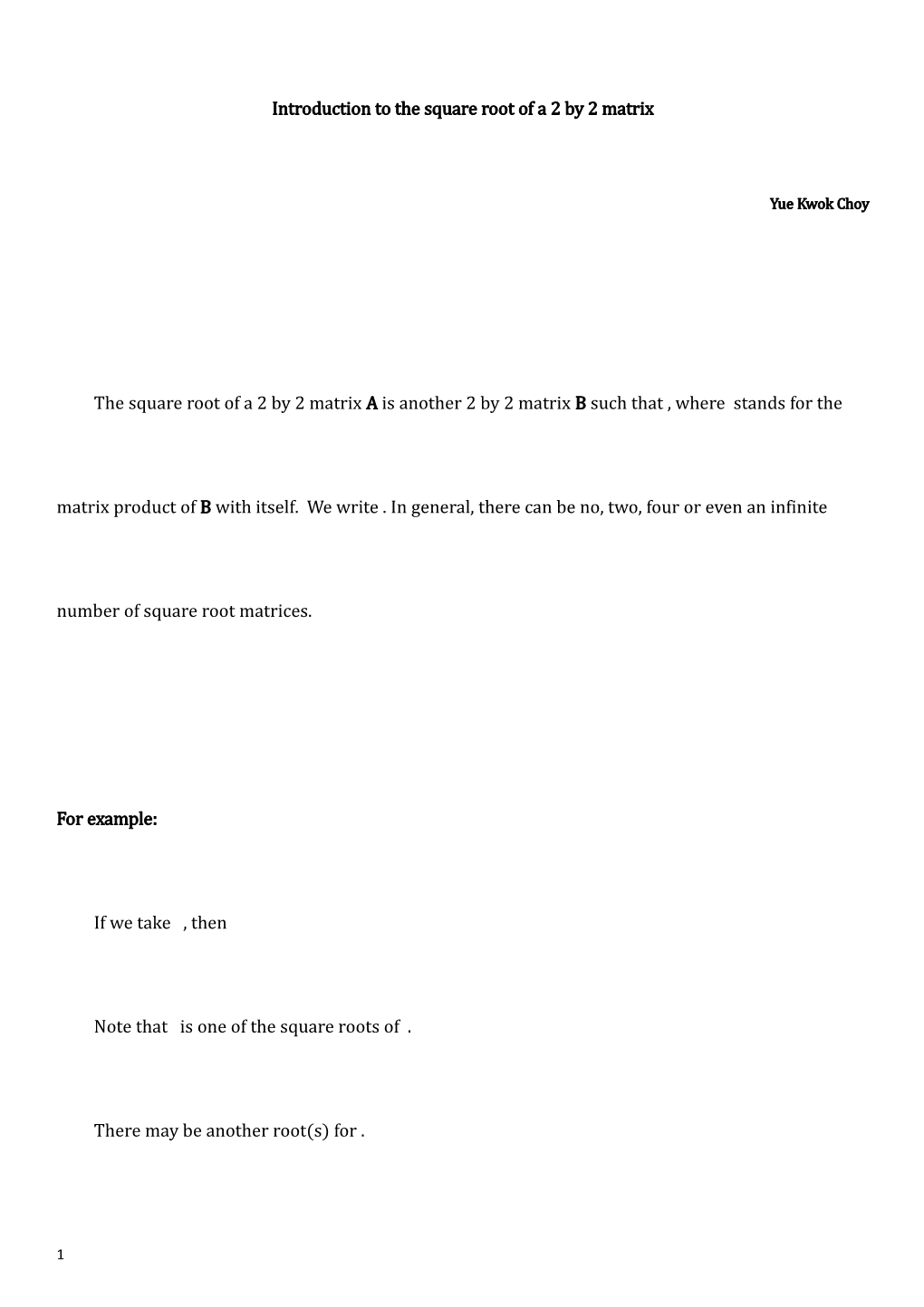# Introduction to the Square Root of a 2 by 2 MatrixIntroduction to the square root of a 2 by 2 matrix

Yue Kwok Choy

The square root of a 2 by 2 matrix A is another 2 by 2 matrix B such that, where stands for the matrix product of B with itself. We write . In general, there can be no, two, four or even an infinite number of square root matrices.

For example:

If we take , then

Note that is one of the square roots of .

There may be anotherroot(s) for .

Questions (See answers at the end of this file)

1.Evaluate : . Hence find two square roots of (a)(b) .

2.Find four values of . Hence find four values of , where .

3.List all possible matrices , where a, b, c and d can be either 0 or 1, such that does not exist.

(A)Solving equations method

Suppose we like to find the square root of . So we write:

We get four equations:

…(4)

Since (otherwise (2) or (3) give absurdity), .

Hence we get:

,

or

The rest can be solved easily, we therefore have:

3.Prove that does not exist.

List, without prove, all possible matrices , where a, b, c and d can be either 0 or 1, such that has no real solution(s).

4.Use algebraic method to find .

5.Check that the square root of the identity matrix is given by:

,

where are limiting cases.

6.(a)Let , find and . Hence find.

(b), where . Given that .

Show that . Hence find .

(B)Diagonalization of Matrrix

Solving equation method in finding the square root of a matrix may not be easy. It involves solving four non-linear equations with four unknowns. You may try this:, and soon may give up.

We note that the square root of a diagonal matrix can be found easily:

.

If a matrix is NOT a diagonal matrix, we devise a method called diagonalization to help us.

We proceed with the finding of the eigenvalue(s) and eigenvector(s) of A .

A real number  is said to be an eigenvalue of a matrix A if there exists a non-zero column vector v such that A.

We like to find

(1)Eigenvalues

Now, has non-zero solution,

, and these are the eigenvalues.

(2)Eigenvectors

We usually would like to find the eigenvector corresponding to each eigenvalue.

The process is called normalization.

For ,

Choose for convenience

 , which is a eigenvector.

For ,

Choose

 , which is another eigenvector.

(3)Diagonalization of matrix

We place two eigenvectors together. Let

Consider another matrix:

At last we get a matrix B which is diagonal with eigenvalues as entries in the main diagonal.

Note:

The diagonalization of a matrix may not be a simple subject since may have equal roots or even complex roots. Although most matrices are not diagonal, they can be diagonalized. Not all square matrices can be diagonalised.A thorough study of diagonalization of a matrix is not discussed here.

(4)Finding the square root of the original matrix A

Since , we have

So

(a)

(b)

Questions

7.Real matrix may have irrational square roots. Check by multipication:

8.A simple real matrix may have complex square roots. Check by multipication:

9.A matrix can have both integral and fractional square roots. Use Diagonalization Method to show:

1. , Hence:

(a)

(b)

2.

3.

(a) Since and so .

(b) Lastly, since and so , contradicts with (a).

does not exist

has no real solution(s).

4.

We get four equations:

…(4)

From (3), ( gives contradiction in (2))

From (1), .

From (3),

Hence,

6.(a), , .

Hence . .

(b),

, by (1).

, where .

Hence, .

9.Eigenvalues : 4, 1

Eigenvectors:

,

(a)

(b)

1